Next: Spherical Harmonics Up: Potential Theory Previous: Introduction

# Associated Legendre Functions

The associated Legendre functions,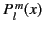, are the well-behaved solutions of the differential equation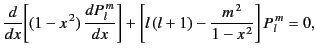(292)

for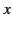in the range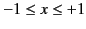. Here,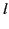is a non-negative integer (known as the degree), and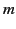is an integer (known as the order) lying in the range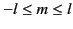. The functions themselves take the form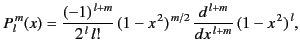(293)

which implies that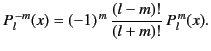(294)

Assuming that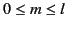, thesatisfy the orthogonality condition(295)

where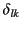is a Kronecker delta symbol.

The associated Legendre functions of order 0 (i.e.,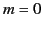) are called Legendre polynomials, and are denoted the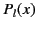: that is,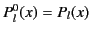. It follows that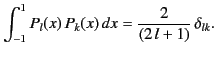(296)

It can also be shown that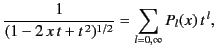(297)

provided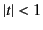and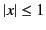.

All of the associated Legendre functions of degree less than 3 are listed below: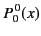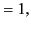(298)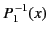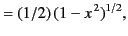(299)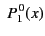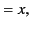(300)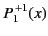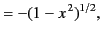(301)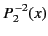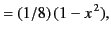(302)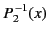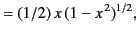(303)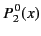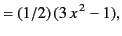(304)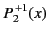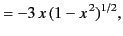(305)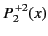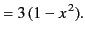(306)Next: Spherical Harmonics Up: Potential Theory Previous: Introduction
Richard Fitzpatrick 2014-06-27